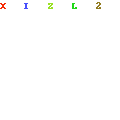# PEMDAS and the Common Errors in the Order of Operations

PEMDAS is the acronym for the order of operations. P stands for parentheses, E for exponent, and MDAS for Mulitplication, Division, Addition, and Subtraction respectively.This means we want to evaluate a numerical expression such as$5(6 + 3)^2 - 8$

we have to ‘operate’ to the order of PEMDAS. The operation is as follows.

Parentheses:$5(6 + 3)^2 - 8 = 5(9)^2 - 8$.

Exponent:$5(9)^2 - 8 = 5(81) - 8$

Multiplication:$5(81) - 8 = 405 - 8$

Subtraction:$405 - 8 = 397$

Now, the common misconception in PEMDAS is when the order of operations MD are adjacent. Since M comes before D, it is thought that M should always be performed first. This is not the case.When multiplication and division are adjacent operations, the operation should be performed from left to right. For example,$8 \div 4 \times 3 = 6$ and not$\frac{8}{12}$ since we have to divide first before we multiply.

This is also similar with addition and subtraction. If the two operations are adjacent, it should be performed from left to right.  The answer to$5 - (-3) + 7 = 15$

and not$5 - (-3) + 7 = 5 - 4 = 1$

With that said, what is the answer to$6 \div 2(1 + 2)$? Is it equal to$9$ or is it equal to$1$?